# C++ Program to Check Armstrong Number

In this tutorial you will learn about the C++ Program to Check Armstrong Number and its application with practical example.

## C++ Program to Check Armstrong Number

In this tutorial, we will learn to create a C++ program that will Check Armstrong Number in C++ programming.

## Prerequisites.

Before starting with this tutorial we assume that you are best aware of the following C++ programming topics:

• Operators in C++ Programming.
• Basic Input and Output function in C++ Programming.
• Basic C++ programming.
• While loop in C++ programming.

## Program to Check Armstrong Number:–

In today’s tutorial, we will create a program that will find the Armstrong numbers from the given number. First will take the number from the user and then will find the Armstrong Number.

With the help of this program, we can Check Armstrong Number.

## Output:-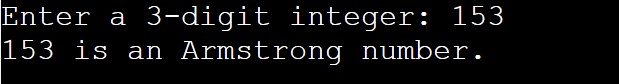In the above program, we have first initialized the required variable.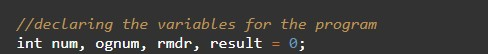• ognum = it will hold the integer value.
• rmdr = it will hold the integer value.
• result = it will hold the integer value.
• num = it will hold the integer value.

Taking the input integer number from the user.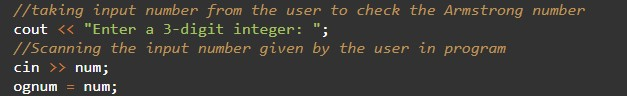Checking the Armstrong numbers.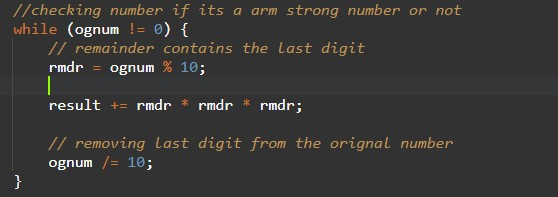Printing the output.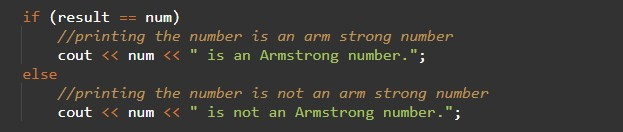In this tutorial we have learn about the C++ Program to Check Armstrong Number and its application with practical example. I hope you will like this tutorial.